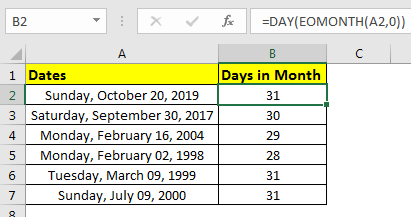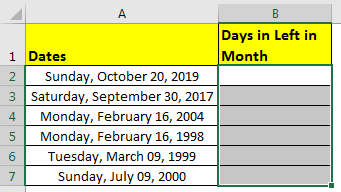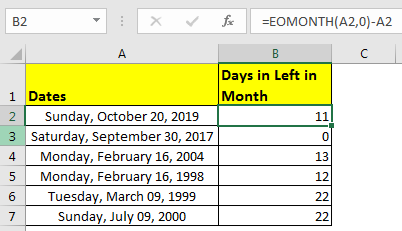# How to Calculate Remaining Days of Month in ExcelPreviously, we learned how to get number of days in a month using a given date. In this article, we will learn how to calculate remaining days in month of a given date.

Generic Formula

=EOMONTH(date,0)- date

Date = the date from which you want calculate remaining days in month.
Let’s learn this by an example:

Example: Get Days Remaining Before End of Month
Here I have some dates. Let’s get the days left before the end of month.Write this formula in B2 and drag it down.

=EOMONTH(A2,0)- A2

This will return the days left till end of that date’s month.How it works?
We needed to get the last date of given date’s month and then subtract the date.
We got the last date of the month using Excel EOMONTH function. This returns the serial number of the last date of the month. Then we subtract the original date. The remaining value is the remaining days in month.
So yeah guys, this how you can get number of days remaining in a month of given date in excel. Let me know if you have any doubt or query regarding this article or another excel topic. The comments section is open for you. We will be happy to help you.

## Users are saying about us...

1.Thanks mate! It helped.

Terms and Conditions of use

The applications/code on this site are distributed as is and without warranties or liability. In no event shall the owner of the copyrights, or the authors of the applications/code be liable for any loss of profit, any problems or any damage resulting from the use or evaluation of the applications/code.